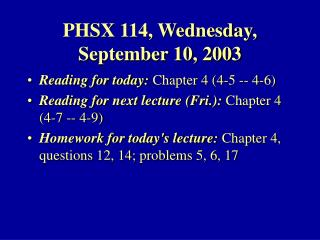# PHSX 114, Wednesday, September 10, 2003 - PowerPoint PPT PresentationDownload PresentationPHSX 114, Wednesday, September 10, 2003

PHSX 114, Wednesday, September 10, 2003Download Presentation## PHSX 114, Wednesday, September 10, 2003

- - - - - - - - - - - - - - - - - - - - - - - - - - - E N D - - - - - - - - - - - - - - - - - - - - - - - - - - -
##### Presentation Transcript

1. PHSX 114, Wednesday, September 10, 2003 • Reading for today: Chapter 4 (4-5 -- 4-6) • Reading for next lecture (Fri.): Chapter 4 (4-7 -- 4-9) • Homework for today's lecture: Chapter 4, questions 12, 14; problems 5, 6, 17

2. Last time: Newton's first and second laws of motion • First law: What happens when there is no net force? • Second law: What happens when there is a net force?

3. Your turn An object has mass 2 kg and two forces act upon it. F1= 3 N in the +x direction, F2= 4 N in the +y direction. Find the magnitude and direction of the acceleration vector. Answer: 2.5 m/s2 , 53.1 degrees from the x direction ax=( F1x+ F2x)/m=(3 + 0)/2 = 1.5 m/s2 , ay=( F1y+ F2y)/m=(0 + 4)/2 = 2 m/s2

4. A force is applied by another object in the environment • Two basic types: 1) contact, 2) action-at-a distance (fundamental forces are of second type) • Newton's 3rd law discusses the relation between the object feeling the force and the object applying the force

5. Here's a stupid question that the first two laws don't address: • Why can't I push myself across the floor?

6. Newton's Third Law of Motion • Whenever one object exerts a force on a second object, the second object exerts a force on the first object that is equal in magnitude and opposite in direction. • Action-reaction • Example: woman pulling sled

7. Introducing two forces • Force of Earth's gravity on an object • Contact force from a surface

8. Weight vs. mass • FG=mg • Weight is a vector (units of Newtons or pounds) • Mass is a scalar (units of kilograms) • An object with a mass of 1 kg has a weight of 9.8 N (downward) • Note: m here is "gravitational mass"

9. Projectile motion and Newton's laws • ΣF=ma • For projectile motion, only force is FG=mg • ΣF=ma=mg • a=g; ax=0, ay=-9.8 m/s2

10. Contact forces from a surface • Normal force, FN, is perpendicular to the surface • Frictional force, Ffr, is along the surface • For today, we ignore friction, focus on normal force

11. Consider a block on a horizontal surface • Examples

12. Your turn • A 20-kg block is sitting on the table. You push down on the block with a force of 30 N. What is the normal force exerted by the table on the block? • Answer: 226 N • FN= 30 N + (20 kg) (9.8 m/s2) = 30 N + 196 N = 226 N

13. Object on a vertical rope • ΣF=ma= FT – FG • Elevator example • FG=mg doesn't change with elevator's acceleration • Tension in rope, FT, determines elevator's acceleration • If a=0 (elevator has constant speed), FT = FG • If a>0 (elevator accelerating upward), FT > FG • If a<0 (elevator accelerating downward), FT < FG